## Introduction

Structures such as external pipeline, fuselage and blade in aeronautical and astronautical engineering play an important role in transfering fuel oil, protecting passengers and providing power and so forth (Barry, 1998). The single type consists of only one component or several components in series, while multiple type include two or more elements connected by clamps or connections. Due to the dynamic load excitations of rotor vibration, fluid pulsation and oscillating combustion during their working process, these engineering structures are prone to have breathing cracks which would seriously affect the integrity and reliability of structures (Timashev and Bushinskaya, 2016). For example, the hydraulic pipe of one aircraft engine resulted in an aircraft fuel tank fire due to resonance fracture and oil leakage, one accident was caused by the crack and leakage of a turbojet engine oil pipe due to impact oscillation. Therefore, in order to ensure the sustainable and stable operation of aeronautical and astronautical engineering structures, and to avoid major safety accidents, it is necessary to identify the structural breathing cracks as early as possible.

For the detection and localization of breathing cracks in engineering structures, numerous methods monitoring changes in static or dynamic structural features caused by cracks have been proposed and applied (Balageas et al., 2010; Kourehli, 2015). To detect and measure cracks, an auto inspection system extracting crack information using Charged Couple Device (CCD) camera and image processing technique has been developed (Yu et al., 2007). Using photographic measurements and wavelet transform algorithm, the slope discontinuity of a beam was identified to indicate the existence and position of cracks (Nigam and Singh, 2020). Based on the wave propagation theory, data of cracked pipeline were collected by piezoceramic transducer and processed by applying wavelet packet decomposition. And then, the root-mean-square deviation (RMSD) was defined as an index for cracked pipeline (Du et al., 2017). Similar to this, researchers (Bhalla and Kiong Soh, 2003) utilized the piezoceramic transducer to measure electro-mechanical impedance (EMI) of cracked structures, and applied its real and imaginary parts respectively as crack indicator. Apart from EMI, magneto-mechanical impedance (MMI) was also used for crack identification. Researchers (Zagrai and Çakan, 2008, 2010) utilized the Magneto-Elastic Active Sensor (MEAS) and impedance analyser to measure MMI and observe its changes for damage detection. The fundamental principle of above methods is simple and testing results on laboratory specimens have verified their reliability and effectiveness. However, these methods would be more suitable for simple structure with single crack fault because that the crack position should be known in prior for the installation of measurement instruments. This drawback would limit their further application to other complex engineering structures that may have multiple cracks.

According to previous studies and results, vibration-based features including modal properties (MP), frequency response function (FRF), output spectrum (OS) and transmissibility function (TF) could be used as effective damage indicators for diagnosing engineering structures with multiple breathing cracks (Prime and Shevitz, 1995; Krawczuk and Ostachowicz, 1996). Compared with others, TF would be more sensitive and useful. For example, it is defined as the ratio of outputs between two different structural points and can be computed utilizing outputs directly. It is a function of structural properties such that it fully reflects intrinsic characteristics of structures with a wide range of frequency and it would be more sensitive to changes in sub-structural parameters due to cracks. Treating breathing cracks as internal force applied to the multi-degree-of-freedom (MDOF) structure, properties of TF were summarized to detect faults in a metal panel (Johnson and Adams, 2002). Later, improved damage indexes were defined to investigate conditions of breathing cracks in a rotorcraft woven composite plate (Kess and Adams, 2007) and a rolling tire (Johnson and Adams, 2006) respectively. To improve the sensitivity of damage indicators and eliminate the effects of inputs, output-based weighting factor was added to increase the difference of TFs between intact and damaged structures (Li et al., 2015a,b). Using operational deflection shape (ODS) at excitation and higher harmonic frequencies, transmissibility of operational deflection shape (TODS) was defined and an outlier detector named teager energy operator (TEO) was used for cracks in a cantilever beam (Li, 2018). To reduce the effects of frequency bands on indicators, output data were processed by wavelet transform (WT) and wavelet spectrum-based TF was used for crack identification (Li et al., 2017).

Since structures with breathing cracks behaviour in a nonlinear way, their nonlinear dynamic models could also be descried by the Volterra series. According to this, nonlinear output frequency response function (NOFRF) was used to compute TF and be employed for localizing multiple cracked elements in a MDOF system (Peng et al., 2007). For the cracks in a reactor coolant pump, one NOFRF TF-based methods applying dual harmonic excitations was proposed (Wang and Ma, 2012). Later, similar TF and indicator were applied for crack faults in a hydraulic pipeline (Li et al., 2015a,b). In order to avoid the estimation of each order NOFRF with complex algorithms, method using nonlinear outputs directly was developed (Zhao et al., 2014). Considering NOFRF and higher harmonic response (HHR) together, a novel crack position index (CPI) was defined for the localization of cracks in a single-span double-disc rotor (Liu et al., 2019). Since the second-order output spectrum (SOOS) is more sensitive to crack-induced nonlinearity, a more sensitive SOOS TF-based damage indicator was proposed for breathing crack faults in a cantilever beam (Jing and Li, 2016).

Above TF-based methods provide information of existence and location of cracks. However, one very important issue of these methods is that features from intact structures are required during the derivation of damage indexes. This requirement could affect these methods’ practicability when the state of benchmark structures cannot be evaluated. To overcome this limitation, a novel vibration-based crack localization method for engineering structures without reference is proposed in this paper. The significant novelties of this paper have, (1) Using outputs of damaged chain-type engineering structures solely, local damage features are derived utilizing additional masses; (2) With the TF-based damage indexes, a novel approach is proposed and its availability is illustrated through simulations on the chain-type MDOF model with multiple breathing cracks.

This paper has five main parts. INTRODUCTION presents the background, some related crack localization methods and the main motivations of this paper. The second part introduces the general chain-type MDOF model of cracked engineering structures and analyses of its dynamic characteristics. The next part derives local damage features and indexes, and summarizes procedures of the novel method. Results of simulation studies are shown in the SIMULATIONS. Finally, conclusions and discussions of this paper are presented in the last part.

## Dynamics of engineering structures

### Model of engineering structure

In some engineering structures such as external pipelines and beams, there is no connection between the head and end of structures such that they are referred to as open chain type structures (Figure 1). To consider this type of structures, their dynamic model is simplified as a general chain-type MDOF model composed of some mass-damper-spring elements (Ewins, 2009).

### Engineering structures with cracks.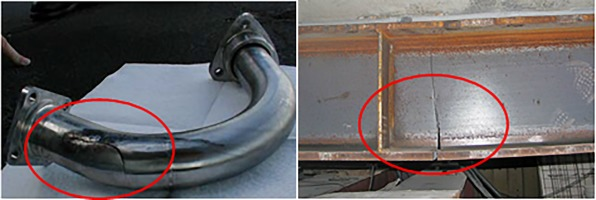As shown in Figure 2, the MDOF model uses coordinate xi(t)(1<i<n) to describe the horizontal motion of each mass, and the single excitation u(t) is applied to the first mass mi. With the Lagrange equation method, the differential equations of motion of this model can be obtained (Ewins, 2009), as

##### (1)
[M]nxn{x¨i(t)}nx1+[C]nxn{x˙i(t)}nx1+[K]nxn{xi(t)}nx1={ui(t)}nx1+{nui(t)}nx1
where M, [C] and [K] are structural mass, damping and stiffness matrices respectively. {x¨i(t)}, {x˙i(t)} and {xi(t)} are structural acceleration, velocity and displacement vectors respectively. {ui(t)} and {nui(t)} are excitation input and nonlinear restoring force vectors respectively.

### Model of engineering structures with cracks.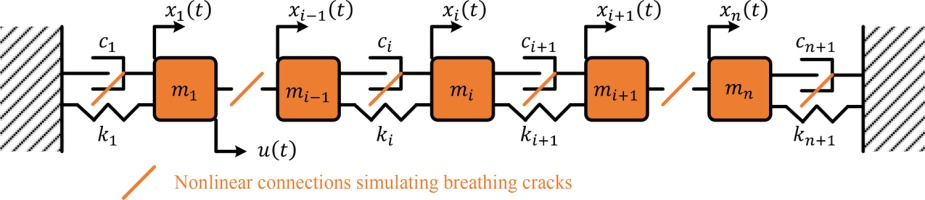If there are breathing cracks in engineering structures, nonlinearities are introduced into damper-spring connecting elements in the MDOF model. Thus, the nonlinear restoring force caused by cracks between masses mi1 and mi+1 could be expressed as a nonlinear function of related output displacements and velocities (Zhao et al., 2014), as

##### (2)
nui(t)=l=2L(nci,ls˙i1,il(t)+nki,lsi1,il(t))+l=2L(nci+1,ls˙i,i+1l(t)+nki+1,lsi,i+1l(t))s˙i,i+1l=(x˙i(t)x˙i+1(t))l,si,i+1l=(xi(t)xi+1(t))l,(1in)

where nci,l and nki,l are nonlinear structural damping and stiffness parameters respectively, L is the maximum order of nonlinear restoring force. If there is no fault around mass mi, corresponding nonlinear structural parameters (nci,l and nki,l) and nonlinear force nui(t) would be close to zero.

### Dynamic response of the MDOF structure

When the excitation in (1) is a cosine function, the output response of mass mi can be expressed with the harmonic balance method (HBM) (Liu et al., 2006), as

##### (3)
xi(t)=v=1V(Aiv(jvω)ejvωt+Biv(jvω)ejvωt)

where V is the maximum order of harmonics, Aiv(jvω) and Biv(jvω) are the vth order complex coefficients.

Substituting (3) into (1) and (2), and extracting the coefficients of ejvωt obtain

##### (4)
[(vω)2M+jvωC+K]nxn{Biv(jvω)}nx1={NUiv(jvω)}nx1(v2)

where {NUiv(jvω)} is the frequency domain nonlinear force vectors caused by cracks, v2 means that only nonlinear output spectrum is considered.

With the matrix [(vω)2M+jvωC+K]1, nonlinear output spectra can be obtained from the following equation.

##### (5)
{Biv(jvω)}nx1=[(vω)2M+jvωC+K]nxn1{NUiv(jvω)}nx1(v2)

It can be seen that nonlinear output spectra at higher harmonic frequencies (more than two) are just decided by structural physical parameters and nonlinear forces induced by cracks.

## Crack localization approach

### Damage features and indicators

Considering mass mi(2in1) in the damaged structure only, and extracting its differential EOM from (4) give

##### (6)
((vω)2mi+jvωci+ki+jvωci+1+ki+1)Biv(jvω)(jvωci+ki)Bi1v(jvω)(jvωci+1+ki+1)Bi+1v(jvω)=NUiv(jvω)(v2)

Now, if additional mass ma is added to mass m1 and excite the structure, equation similar to (6) could be obtained, as

##### (7)
((vω)2mi+jvωci+ki+jvωci+1+ki+1)Biv,a(jvω)(jvωci+ki)Bi1v,a(jvω)(jvωci+1+ki+1)Bi+1v,a(jvω)=NUiv,a(jvω)(v2)

Adding mass mb to mass mn and excite the structure again, another equation similar to (6) and (7) could be obtained, as

##### (8)
((vω)2mi+jvωci+ki+jvωci+1+ki+1)Biv,b(jvω)(jvωci+ki)Bi1v,b(jvω)(jvωci+1+ki+1)Bi+1v,b(jvω)=NUiv,b(jvω)(v2)

Observing (6), (7) and (8), it is found that the change of value of mass m1 or mn leads to variations in output spectra and nonlinear force, while structural parameters around mass mi(2in1) remain constant.

Writing (6), (7) and (8) into a matrix form gives

##### (9)
[BBi1,i,i+1v(jvω)]3x3{QQiv(jvω)}3x1={NNUiv(jvω)}3x1(v2)

where

[BBi1,i,i+1v(jvω)]3x3=[Biv(jvω)Bi1v(jvω)Bi+1v(jvω)Biv,a(jvω)Bi1v,a(jvω)Bi+1v,a(jvω)Biv,b(jvω)Bi1v,b(jvω)Bi+1v,b(jvω)],
{QQiv(jvω)}3x1={((vω)2mi+jvωci+ki+jvωci+1+ki+1)(jvωci+ki)(jvωci+1+ki+1)},
{NNUiv(jvω)}3x1={NUiv(jvω)NUiv,a(jvω)NUiv,b(jvω)}.

It is shown that if there is no fault around mass mi, vector {NNUiv(jvω)} would be zero which in turn makes the matrix [BBi1,i,i+1v(jvω)] be singular. On the contrary, if there is fault around mass mi, vector {NNUiv(jvω)} is nonzero, the matrix [BBi1,i,i+1v(jvω)] should be non-singular and the inverse of matrix [BBi1,i,i+1v(jvω)] exists. Therefore, the singularity of matrix [BBi1,i,i+1v(jvω)] could be a sensitive feature for detecting damages around mass mi.

To determine the singularity of matrix [BBi1,i,i+1v(jvω)], the rank of matrix is a useful calculation method. Thus, a novel damage indicator is defined, as

##### (10)
DIi(jvω)=Rank([BBi1,i,i+1v(jvω)])(2in1,v2)

According to the analysis above, result DIi(jvω)=3 shows that the matrix is full rank and crack may be located at the left and/or right side of mass mi, while result DIi(jvω)<3 shows that the matrix is singular and there is no fault around mass mi.

### Procedures of Method

Based on the damage indicator in (10), a novel crack localization approach is developed. Its procedures are outlined and explained as follows.

Developed method includes four main steps. In steps 1 and 2, time domain output data collected from the engineering structure under estimation are processed and transformed into frequency domain to form matrix [BBi1,i,i+1v(jvω)]. In step 3, the rank of matrix [BBi1,i,i+1v(jvω)] is calculated and regarded as the damage indicator. In the final step, multiple faults are detected according to the value of indicator DIi(jvω)

## Simulations

To verify the effectiveness of above damage indicator and localization method, two simulation cases on the chain type structure with six DOFs (Figure 3) are studied respectively. In case one, one fault is between masses m1 and m2, and the other fault is between masses m5 and m6. In case two, one fault is between masses m3 and m4, and the other fault is between masses m4 and m5. The simulation would be performed by the Matlab software and program.

#### Schematic diagram of cases, (a) Case one. (b) Case two.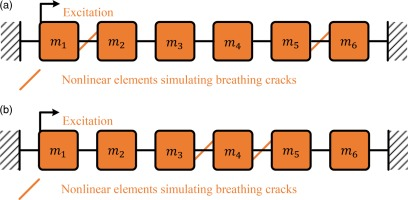Structural parameters of simulation models are shown in Table 1.

#### Parameters of simulation.

Coordinatesi = 1i = 2i = 3i = 4i = 5i = 6i = 7
mass m123456
damping c (1e3)0.360.721.081.441.802.162.52
stiffness k (1e5)1.721.442.161.883.608.322.04
Additional massma = 10; mb = 24
Case onenc1,2 = 520; nk1,2 = 64,000; nc5,2 = 240; nk5,2 = 168,000; Other nc and nk are zero
Case twonc3,2 = 420; nk3,2 = 54,000; nc4,2 = 440; nk4,2 = 111,000; Other nc and nk are zero

According to (2), faults are simulated as nonlinear elements with corresponding nonlinear parameters (nci,2,nki,2). To obtain three similar equations (6), (7) and (8), the input applied to mass m1 is a cosine function u(t)=cos(2πft) with frequency 8 Hz and masses ma=20 and mb=24 are added to masses m1 and m6 in the model respectively.

With the procedures of approach in Figure 4, results of simulation cases are shown in Figure 5.

#### Procedures of localization method.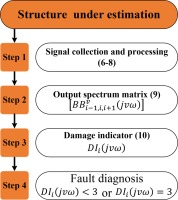#### Results of simulation. (a) Case one. (b) Case two.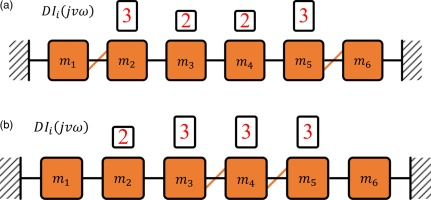It can be seen that values of DI2(jvω) and DI5(jvω) are three while values of DI3(jvω) and DI4(jvω) are two in case one, which indicates that the area round masses m3 and m4 is free from faults, and faults are at around other masses. Similar to the results in case one, results from case two demonstrate that there is no fault around mass m2 and faults are round masses m4 and m5 since only the value of DI2(jvω) is less than three.

Overall, detected results from chain-type structures are consistent with the crack positions in case setups. Thus, the effectiveness of proposed method is verified by these two simulation cases.

## Results and discussions

To avoid benchmark features from intact structures during the derivation of damage indicators in previous methods. A novel vibration-based crack localization method of engineering structures without reference is proposed in this study. The main conclusions are summarized as follows.

1. By simulating effects of cracks as nonlinear restoring forces, a general MDOF model with nonlinear connecting elements could be used to describe chain-type engineering structures’ dynamic behaviour.

2. Adding additional mass to the structure, three similar equations from structure under estimation could be used to form one matrix, and its rank could be an effective damage indicator to indicate the existence and position of cracks.

3. Results from simulations on the chain-type structures with multiple nonlinear elements (cracks) demonstrate that the proposed approach could be an effective tool to estimate the state of cracked engineering structures.

4. In the future study, experimental verification of this approach and extension of this approach to other kinds of engineering structures should be considered more.

## Nomenclature

[M]

structural mass matrix

[C]

structural damping matrix

[K]

structural stiffness matrix

{x¨i(t)}

structural acceleration vector

{x˙i(t)}

structural velocity vector

{x˙i(t)}

structural displacement vector

{ui(t)}

excitation vector

{nui(t)}

nonlinear restoring force vector

nci,l

nonlinear structural damping parameter

nki,l

nonlinear structural stiffness parameter

L

maximum order of nonlinear restoring force

V

maximum order of harmonics

V

the vth  order complex coefficient

Biv(jvω)

the vth order complex coefficient

{NUiv(jvω)}

frequency domain nonlinear force vector

DIi(jvω)

a novel damage indicator.